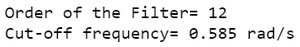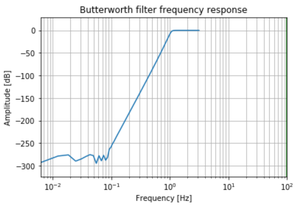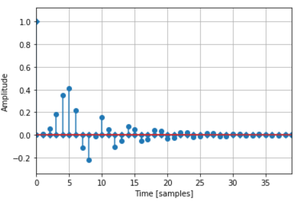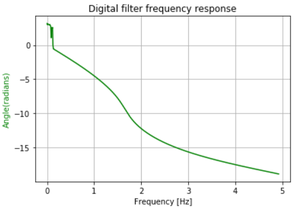# Digital High Pass Butterworth Filter in Python

• Last Updated : 14 Aug, 2021

In this article, we are going to discuss how to design a Digital High Pass Butterworth Filter using Python. The Butterworth filter is a type of signal processing filter designed to have a frequency response as flat as possible in the pass band. Let us take the below specifications to design the filter and observe the Magnitude, Phase & Impulse Response of the Digital Butterworth Filter.

## What is a High Pass Filter?

A high-pass filter is an electronic filter that passes signals with a frequency higher than a certain cutoff frequency and attenuates signals with frequencies lower than the cutoff frequency. The attenuation for each frequency depends on the filter design.

## Difference between a Digital High Pass Filter & Digital Low Pass Filter:

The most striking difference is in the amplitude response of the filters, we can clearly observe that in case of High Pass Filter the filter passes signals with a frequency higher than a certain cutoff frequency and attenuates signals with frequencies lower than the cutoff frequency while in case of Low Pass Filter the filter passes signals with a frequency lower than a certain cutoff frequency and attenuates all signals with frequencies higher than the specified cutoff value.

The specifications are as follows:

• Sampling rate of 3.5 kHz
• Pass band edge frequency of 1050 Hz
• Stop band edge frequency of 600Hz
• Pass band ripple of 1 dB
• Minimum stop band attenuation of 50 dB

We will plot the magnitude, phase, and impulse response of the filter.

Step-by-step Approach:

Step 1: Importing all the necessary libraries.

## Python3

 `# Import required modules ` `import` `numpy as np ` `import` `matplotlib.pyplot as plt ` `from` `scipy ``import` `signal ` `import` `math`

Step 2: Define variables with the given specifications of the filter.

## Python3

 `# Specifications of Filter ` `  `  ` ``# sampling frequency ` `f_sample ``=` `3500` `  `  `# pass band frequency ` `f_pass ``=` `1050` `  `  `# stop band frequency ` `f_stop ``=` `600` `  `  `# pass band ripple ` `fs ``=` `0.5` `  `  `# pass band freq in radian ` `wp ``=` `f_pass``/``(f_sample``/``2``)   ` `  `  `# stop band freq in radian ` `ws ``=` `f_stop``/``(f_sample``/``2``)  ` `  `  `# Sampling Time ` `Td ``=` `1`  `  `  ` ``# pass band ripple ` `g_pass ``=` `1` `  `  `# stop band attenuation ` `g_stop ``=` `50`

Step3: Building the filter using signal.buttord() method.

## Python3

 `# Conversion to prewrapped analog frequency ` `omega_p ``=` `(``2``/``Td)``*``np.tan(wp``/``2``) ` `omega_s ``=` `(``2``/``Td)``*``np.tan(ws``/``2``) ` `  `  `  `  `# Design of Filter using signal.buttord function ` `N, Wn ``=` `signal.buttord(omega_p, omega_s, g_pass, g_stop, analog``=``True``) ` `  `  `  `  `# Printing the values of order & cut-off frequency! ` `print``(``"Order of the Filter="``, N)  ``# N is the order ` `# Wn is the cut-off freq of the filter ` `print``(``"Cut-off frequency= {:.3f} rad/s "``.``format``(Wn)) ` `  `  `  `  `# Conversion in Z-domain ` `  `  `# b is the numerator of the filter & a is the denominator ` `b, a ``=` `signal.butter(N, Wn, ``'high'``, ``True``) ` `z, p ``=` `signal.bilinear(b, a, fs) ` `# w is the freq in z-domain & h is the magnitude in z-domain ` `w, h ``=` `signal.freqz(z, p, ``512``)`

Output:Step 4: Plotting the Magnitude Response.

## Python3

 `# Magnitude Response` `plt.semilogx(w, ``20``*``np.log10(``abs``(h)))` `plt.xscale(``'log'``)`   `plt.title(``'Butterworth filter frequency response'``)` `plt.xlabel(``'Frequency [Hz]'``)` `plt.ylabel(``'Amplitude [dB]'``)` `plt.margins(``0``, ``0.1``)`   `plt.grid(which``=``'both'``, axis``=``'both'``)` `plt.axvline(``100``, color``=``'green'``)` `plt.show()`

Output:Step 5: Plotting the Impulse Response.

## Python3

 `# Impulse response` `imp ``=` `signal.unit_impulse(``40``)` `c, d ``=` `signal.butter(N, ``0.5``)` `response ``=` `signal.lfilter(c, d, imp)`   `# Illustrating impulse response` `plt.stem(np.arange(``0``, ``40``), imp, markerfmt``=``'D'``, use_line_collection``=``True``)` `plt.stem(np.arange(``0``, ``40``), response, use_line_collection``=``True``)` `plt.margins(``0``, ``0.1``)`   `plt.xlabel(``'Time [samples]'``)` `plt.ylabel(``'Amplitude'``)` `plt.grid(``True``)` `plt.show()`

Output:Step 6: Plotting the Phase Response.

## Python3

 `# Phase response` `fig, ax1 ``=` `plt.subplots()`   `ax1.set_title(``'Digital filter frequency response'``)` `ax1.set_ylabel(``'Angle(radians)'``, color``=``'g'``)` `ax1.set_xlabel(``'Frequency [Hz]'``)`   `angles ``=` `np.unwrap(np.angle(h))` `ax1.plot(w``/``2``*``np.pi, angles, ``'g'``)` `ax1.grid()` `ax1.axis(``'tight'``)`   `plt.show()`

Output:Below is the complete program based on the above approach:

## Python3

 `# import required modules ` `import` `numpy as np ` `import` `matplotlib.pyplot as plt ` `from` `scipy ``import` `signal ` `import` `math`     `# Specifications of Filter ` `  `  ` ``# sampling frequency ` `f_sample ``=` `3500` `  `  `# pass band frequency ` `f_pass ``=` `1050` `  `  `# stop band frequency ` `f_stop ``=` `600` `  `  `# pass band ripple ` `fs ``=` `0.5` `  `  `# pass band freq in radian ` `wp ``=` `f_pass``/``(f_sample``/``2``)   ` `  `  `# stop band freq in radian ` `ws ``=` `f_stop``/``(f_sample``/``2``)  ` `  `  `# Sampling Time ` `Td ``=` `1`  `  `  ` ``# pass band ripple ` `g_pass ``=` `1` `  `  `# stop band attenuation ` `g_stop ``=` `50`   `# Conversion to prewrapped analog frequency ` `omega_p ``=` `(``2``/``Td)``*``np.tan(wp``/``2``) ` `omega_s ``=` `(``2``/``Td)``*``np.tan(ws``/``2``) ` `  `  `  `  `# Design of Filter using signal.buttord function ` `N, Wn ``=` `signal.buttord(omega_p, omega_s, g_pass, g_stop, analog``=``True``) ` `  `  `  `  `# Printing the values of order & cut-off frequency! ` `print``(``"Order of the Filter="``, N)  ``# N is the order ` `# Wn is the cut-off freq of the filter ` `print``(``"Cut-off frequency= {:.3f} rad/s "``.``format``(Wn)) ` `  `  `  `  `# Conversion in Z-domain ` `  `  `# b is the numerator of the filter & a is the denominator ` `b, a ``=` `signal.butter(N, Wn, ``'high'``, ``True``) ` `z, p ``=` `signal.bilinear(b, a, fs) `   `# w is the freq in z-domain & h is the magnitude in z-domain ` `w, h ``=` `signal.freqz(z, p, ``512``)`     `# Magnitude Response ` `plt.semilogx(w, ``20``*``np.log10(``abs``(h))) ` `plt.xscale(``'log'``) ` `plt.title(``'Butterworth filter frequency response'``) ` `plt.xlabel(``'Frequency [Hz]'``) ` `plt.ylabel(``'Amplitude [dB]'``) ` `plt.margins(``0``, ``0.1``) ` `plt.grid(which``=``'both'``, axis``=``'both'``) ` `plt.axvline(``100``, color``=``'green'``) ` `plt.show()`     `# Impulse Response` `imp ``=` `signal.unit_impulse(``40``)` `c, d ``=` `signal.butter(N, ``0.5``)` `response ``=` `signal.lfilter(c, d, imp)` `plt.stem(np.arange(``0``, ``40``),imp,markerfmt``=``'D'``,use_line_collection``=``True``)` `plt.stem(np.arange(``0``,``40``), response,use_line_collection``=``True``)` `plt.margins(``0``, ``0.1``)` `plt.xlabel(``'Time [samples]'``)` `plt.ylabel(``'Amplitude'``)` `plt.grid(``True``)` `plt.show()`     `# Phase Response` `fig, ax1 ``=` `plt.subplots()` `ax1.set_title(``'Digital filter frequency response'``)` `ax1.set_ylabel(``'Angle(radians)'``, color``=``'g'``)` `ax1.set_xlabel(``'Frequency [Hz]'``)` `angles ``=` `np.unwrap(np.angle(h))` `ax1.plot(w``/``2``*``np.pi, angles, ``'g'``)` `ax1.grid()` `ax1.axis(``'tight'``)` `plt.show()`

Output:My Personal Notes arrow_drop_up
Recommended Articles
Page :